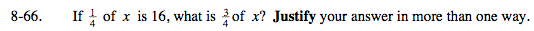### Home > MC2 > Chapter 8 > Lesson 8.2.4 > Problem8-66

8-66.Write out equations to represent these phrases.

$\text{If }\frac{1}{4}x = 16,\text{ find } \frac{3}{4}x.$

Solve for x in the first equation: x = 64

Substitute 64 for x in the second equation.

$\frac{3}{4} (64)$